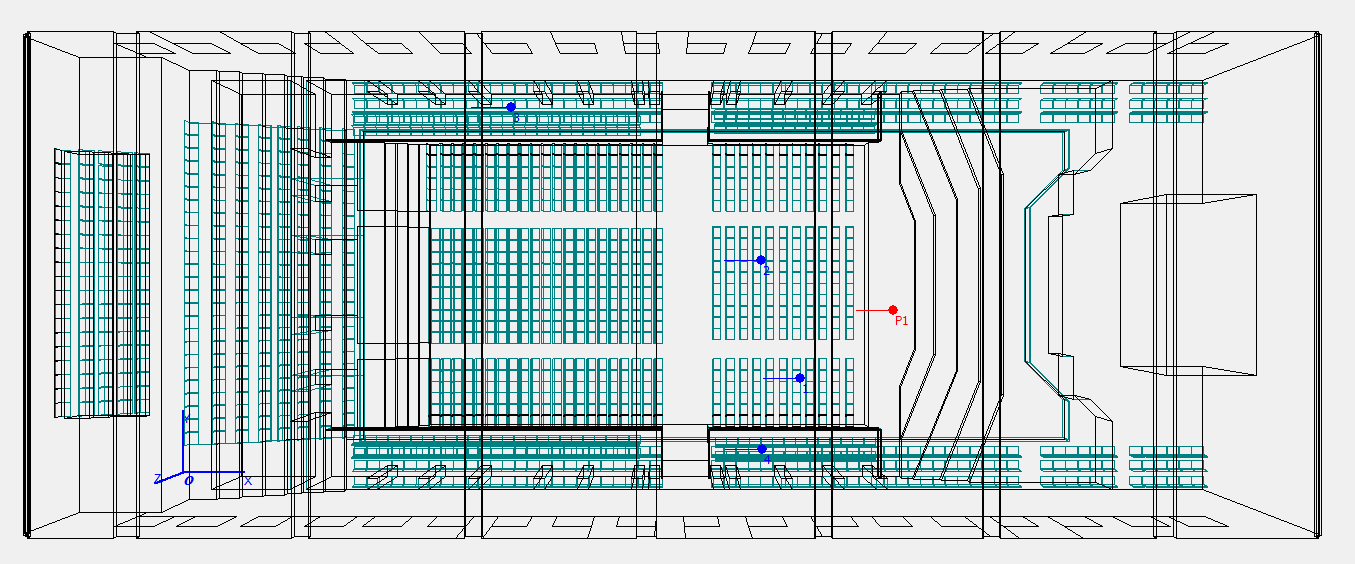# Accompanying webpage for our ISMRA 2016 paper

This is the accompanying website for the conference paper entitled "Finite difference room acoustics simulation with general impedance boundaries and viscothermal losses in air: Parallel implementation on multiple GPUs", presented at the International Symposium on Room and Musical Acoustics (ISMRA), in La Plata, Argentina, September, 2016 (). Download the paper here.

## CUDA Kernels

The CUDA kernels used for Equation (11), and Equations (12) and (8b) and (8c) in  are found below. Please see the paper () for the referenced equations.

In these CUDA kernels, `ReaL` is hash-defined to either `float` or `double`, and `u,u1,u2`, each of size `Nx*Ny*Nz`, represent the grid function Ψ respectively at times: `n+1, n, n-1`. Undeclared variables reside in constant memory or are hash-defined. All of the kernels use a maximum-threading approach (see  for a detailed explanation).

This CUDA kernel implements Equation (11) in . Each thread operates over one point in a 3D grid of size `Nx*Ny*Nz`.

``````  __global__ void Kernel1(ReaL *u, const ReaL * __restrict__ u1, const ReaL * __restrict__ u2, char *Ki) {
int x = blockIdx.x*Bx + threadIdx.x;
int y = blockIdx.y*By + threadIdx.y;
int z = blockIdx.z*Bz + threadIdx.z;
if ((x<Nx) && (y<Ny) && (z<Nz)) {         //if not outside allocated memory
unsigned int cp = z*Ny*Nx + y*Nx + x;  //calculate linear index
int K = Ki[cp];                        //get K value
if (K>0) {                             //if inside domain
ReaL Q1 = u1[cp+1]+u1[cp-1]+u1[cp+Nx]+u1[cp-Nx]+u1[cp+Nx*Ny]+u1[cp-Nx*Ny];
ReaL Q2 = u2[cp+1]+u1[cp-1]+u2[cp+Nx]+u2[cp-Nx]+u2[cp+Nx*Ny]+u2[cp-Nx*Ny];
u[cp] = (2.0-(l2+l2taup)*K)*u1[cp] + (l2taup*K-1.0)*u2[cp] + (l2+l2taup)*Q1 - l2taup*Q2;
}
}
}
``````

This CUDA kernel implements Equation (12) in  in the frequency-independent case, with each thread operating over one of `Nb` boundary points.

``````  __global__ void Kernel2A(ReaL *u, ReaL *u2, unsigned int *Ib, char *KbarIb, char *MIb) {
int bb = blockIdx.x*Bb + threadIdx.x;
if (bb<Nb) {                              //if not outside allocated memory for Ib
unsigned int cp = Ib[bb];              //get linear index of grid value
int mi = MIb[bb];                      //get material index at boundary node
ReaL hlKbB = 0.5*l*KbIb[bb]*beta[mi];  //0.5 * lambda * K-bar * beta at boundary node
u[cp] = (u[cp] + hlKbB*u2[cp])/(1.0+hlKbB);
}
}
``````

This CUDA kernel implements Equations (12) and (8b) and (8c) in  in the frequency-dependent case, with each thread operating over one of `Nb` boundary points.

``````  __global__ void Kernel2B(ReaL *u, ReaL *u2, ReaL *v1, ReaL *v2, ReaL *g1, unsigned int *Ib, char *KbarIb, char *MIb) {
int bb = blockIdx.x*Bb + threadIdx.x;
if (bb<Nb) {                              //if not outside allocated memory for Ib
unsigned int cp = Ib[bb];              //get linear index of grid value
int mi = MIb[bb];                      //get material index at boundary node
ReaL lKbar = l*KbarIb[bb];             //lambda * K-bar value at boundary node
ReaL ucp = u[cp];                      //read u from global memory
ReaL u2cp = u2[cp];                    //read u2 from global memory
ReaL g1cpb[Mb],v1cpb,v2cpb[Mb];
unsigned int cpb;
for(int m=0;m<Mb;m++) {                //loop over max Mb branches
cpb = m*Nb + bb;                    //get linear index of extra boundary values
g1cpb[m] = g1[cpb];                 //read g1 from global memory
v2cpb[m] = v2[cpb];                 //read v2 from global memory
ucp = ucp-(lKbar*bi[mi][m]*(2.0*D[mi][m]*v2cpb[m] - F[mi][m]*g1cpb[m]));
}
ucp=(ucp + 0.5*lKbar*beta[mi]*u2cp)/(1.0+0.5*lKbar*beta[mi]);
u[cp]=ucp;                             //write u to global memory
for(int m=0;m<Mb;m++) {                //loop over max Mb branches
cpb = m*Nb + bb;                    //get linear index of extra boundary values
v1cpb = bi[mi][m]*((ucp-u2cp) + di[mi][m]*v2cpb[m]-2.0*F[mi][m]*g1cpb[m]);
g1[cpb] = g1cpb[m]+0.5*(v1cpb+v2cpb[m]);  //write g1 to global memory
v1[cpb] = v1cpb;                          //write v1 to global memory
}
}
}
``````

## Sound examples

These are sound examples resulting from the concert hall model simulation run over four Nvidia K20 GPU devices. Here, a dry cello sound is convolved with impulse responses that are bandlimited to 4kHz:

R1:

R2:

R3:

R4:

The codes R1--R4 correspond to different receiver locations, all with one fixed source position (see the figures below). The dry cello sample was taken from ODEON's website.

The simulated impulse responses (bandlimited to 4kHz) can be heard on their own here:

R1:

R2:

R3:

R4:

Keep in mind, this is an incomplete and highly bandlimited FDTD model, so it should not be expected to closely resemble Musikverein's Golden Hall!

## References

 B. Hamilton, C. J. Webb, N. D. Fletcher, and S. Bilbao, Finite difference room acoustics simulation with general impedance boundaries and viscothermal losses in air: Parallel implementation on multiple GPUs. Proceedings of the International Symposium on Room and Musical Acoustics, 2016.

 C. J. Webb, Parallel computation techniques for virtual acoustics and physical modelling synthesis. Ph.D. thesis, University of Edinburgh, 2014.Last updated 11/13/2016.